# The region in the xy -plane defined by the inequality − 1 ≤ y ≤ 3 .### Single Variable Calculus: Concepts...

4th Edition
James Stewart
Publisher: Cengage Learning
ISBN: 9781337687805### Single Variable Calculus: Concepts...

4th Edition
James Stewart
Publisher: Cengage Learning
ISBN: 9781337687805

#### Solutions

Chapter T, Problem 5BDT

(a)

To determine

## To sketch: The region in the xy-plane defined by the inequality −1≤y≤3.

Expert Solution

### Explanation of Solution

The graph of the region in the xy-plane defined by the inequality 1y3 is sketched below in Figure 1,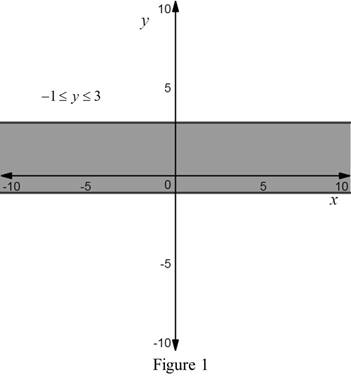In Figure 1, the region in the xy-plane defined by the inequality 1y3 is shaded.

(b)

To determine

Expert Solution

### Explanation of Solution

The graph of the region in the xy-plane defined by the inequalities |x|<4 and |y|<2 is sketched below in Figure 2,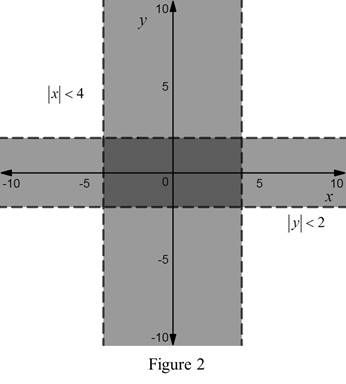In Figure 2, the region in the xy-plane defined by the inequalities |x|<4 and |y|<2 is shaded.

(c)

To determine

Expert Solution

### Explanation of Solution

The graph of the region in the xy-plane defined by the inequality y<112x is sketched below in Figure 3,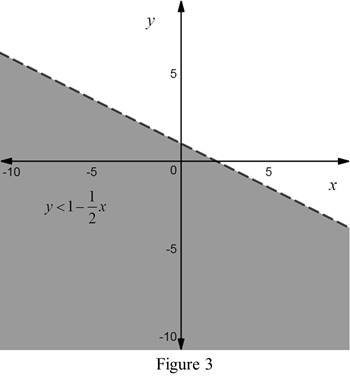In Figure 3, the region in the xy-plane defined by the inequality y<112x is shaded.

(d)

To determine

Expert Solution

### Explanation of Solution

The graph of the region in the xy-plane defined by the inequality yx21 is sketched below in Figure 4,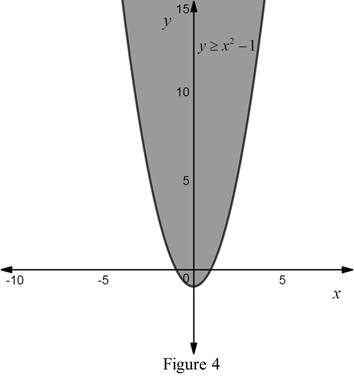In Figure 4, the region in the xy-plane defined by the inequality yx21 is shaded.

(e)

To determine

Expert Solution

### Explanation of Solution

The graph of the region in the xy-plane defined by the inequality x2+y2<4 is sketched below in Figure 5,In Figure 5, the region in the xy-plane defined by the inequality x2+y2<4 is shaded.

(f)

To determine

Expert Solution

### Explanation of Solution

The graph of the region in the xy-plane defined by the equation 9x2+16y2=144 is sketched below in Figure 6,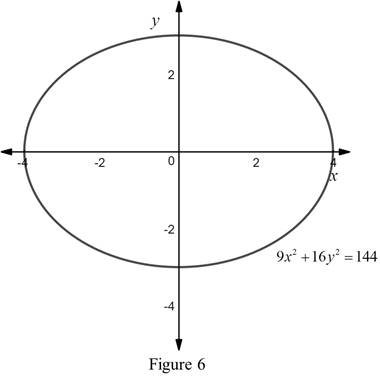In Figure 6, the region in the xy-plane defined by the equation 9x2+16y2=144 is shaded.

### Have a homework question?

Subscribe to bartleby learn! Ask subject matter experts 30 homework questions each month. Plus, you’ll have access to millions of step-by-step textbook answers!